Support
Technical Support

# Calculation Formulas

View: 813  From: HONG'S BELT  Date: 2013-01-06

Units Conversion

 English (USA) Unit X Multiply by = Metric Unit X Multiply by = English (USA) Unit Linear Measure in 25.40 mm 0.0394 in Linear Measure in 0.0254 m 39.37 in ft 304.8 mm 0.0033 ft ft 0.3048 m 3.281 ft Square Measure in2 645.2 mm2 0.00155 in2 Square Measure in2 0.000645 m2 1550.0 in2 ft2 92.903 mm2 0.00001 ft2 ft2 0.0929 m2 10.764 ft2 Cubic Measure ft3 0.0283 m3 35.31 ft3 Cubic Measure ft3 28.32 L 0.0353 ft3 Speed Rate ft / s 18.29 m / min 0.0547 ft / s Speed Rate ft / min 0.3048 m / min 3.281 ft / min Avoirdupois Weight lb 0.4536 kg 2.205 lb Avoirdupois Weight lb / ft3 16.02 kg / m3 0.0624 lb / ft3 Bearing Capacity lb 0.4536 kg 2.205 lb Bearing Capacity lb 4.448 Newton (N) 0.225 lb kg 9.807 Newton (N) 0.102 kg lb / ft 1.488 kg / m 0.672 lb / ft lb / ft 14.59 N / m 0.0685 lb / ft kg - m 9.807 N / m 0.102 kg - m Torque in - lb 11.52 kg - mm 0.0868 in - lb Torque in - lb 0.113 N - m 8.85 in - lb kg - mm 9.81 N - mm 0.102 kg - mm Rotate Inertia in4 416.231 mm4 0.0000024 in4 Rotate Inertia in4 41.62 cm4 0.024 in4 Pressure /Stress lb / in2 0.0007 kg / mm2 1422 lb / in2 Pressure / Stress lb / in2 0.0703 kg / cm2 14.22 lb / in2 lb / in2 0.00689 N / mm2 145.0 lb / in2 lb / in2 0.689 N / cm2 1.450 lb / in2 lb / ft2 4.882 kg / m2 0.205 lb / ft2 lb / ft2 47.88 N / m2 0.0209 lb / ft2 Power HP 745.7 watt 0.00134 HP Power ft - lb / min 0.0226 watt 44.25 ft - lb / min Temperature °F T C = ( °F - 32 ) / 1.8 Temperature

Symbol of B D E F

Symbol

Unit

BS

Conveyor Belt Tensile Strength

Kg/M

BW

Belt Width

M

C  Symbol Definition

 Symbol Unit Ca See the Table FC ---- Cb See the Table FC ----

Symbol Definition

Symbol

Unit

DS

Shaft Deflection Ratio

mm

Symbol Definition

Symbol

Unit

E

Shaft Elongation Rate

Gpa

Symbol Definition

Symbol

Unit

FC

Friction Coefficient Between Belt Edge and Hold Down Strip

----

FBP

Friction Coefficient Between Carry Product and Belt Surface

----

FBW

Friction Coefficient of Belt Support Material

----

FA

Coefficient Amended

----

FS

Tensile Strength Coefficient Amended

----

FT

Conveyor Belt Temperature Coefficient Amended

---

Symbol of H I L M

 Symbol Unit H Elevation Conveyor incline altitude. m HP Horsepower HP I   Symbol Definition Symbol Unit I Moment Of Inertia mm4 L  Symbol Definition Symbol Unit L Conveyance Distance (Center Point From Drive Shaft To Idler  Shaft) M LR Return Way Straight Run Section Length M LP Carry Way Straight  Run Section Length M M  Symbol Definition Symbol Unit M Spiral Conveyor Layer Level ---- MHP Motor Horsepower HP

Symbol of P R S

 Symbol Unit PP Product Accumulated Measure Area Percentage of Carry Way ---- R  Symbol Definition Symbol Unit R Sprocket Radius mm RO Outside Radius mm rpm Revolutions Per Minute rpm S  Symbol Definition Symbol Unit SB Interval Between Bearing mm SL Shaft Total Loading Kg SW Shaft Weight Kg/M

Symbol of T V W

 Symbol Unit TA Conveyor Belt Unit Allowable Tension Kg/M TB Conveyor Belt Unit Theory Tension Kg/M TL Conveyor Belt Unit Catenary's Sag tension. Kg/M TN Tension Of Section kg/M TS Torque Kg.mm TW Conveyor Belt Unit Total Tension Kg/M TWS Particular Type Conveyor Belt Unit Total Tension Kg/M V  Symbol Definition Symbol Unit V Conveyance Speed M/min VS Theory Speed M/min W  Symbol Definition Symbol Unit WB Conveyor Belt Unit Weight Kg/M2 Wf Accumulated Conveyance Friction Stress Kg/M2 WP Conveyor Belt Carry Product Unit Weight

Pusher And Bidirectional

For the pusher or bidirectional conveyor, the belt tension will be higher than the ordinary horizontal conveyor; therefore, the shafts at two ends are necessary to be considered as drive shafts and subsumed in the calculation. In general, it is approximate 2.2 times the experience factor to get the total belt tension.

 FORMULA : TWS = 2.2 TW = 2.2 TB X FA TWS in this unit means the tension calculation of the bidirectional or pusher conveyor.

Turning Calculation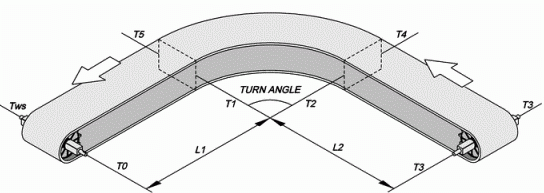The tension calculation TWS of the turning conveyor is to calculate the accumulated tension. Therefore, the tension in every carrying section will affect the value of total tension. That means, the total tension is accumulated from the the beginning of the drive section in return way, along the return way to the idler section, and then pass through the carrying section to the drive section.

The design point in this unit is T0 that under the drive shaft. The value of T0 is equal to zero; we calculate every section from T0. For example, the first straight section in return way is from T0 to T1, and that means the accumulated tension of T1.

T2 is the accumulated tension of the turning position in the return way; in another word, it is the accumulated tension of T0, T1 and T2. Please according to the illustration above and figure out the accumulated tension of the latter sections.

 FORMULA : TWS = ( T6 ) Total tension of the drive section in the carrying way. TWS in this unit means the tension calculation of the turning conveyor. FORMULA : T0 = 0 T1 = WB + FBW X LR  X WB Tension of catenary sag at the drive position. FORMULA : TN = ( Ca X TN-1 ) + ( Cb X FBW X RO ) X WB Tension of the turning section in the return way. For the value Ca and Cb, please refer to Table Fc. T2 = ( Ca X T2-1 ) + ( Cb X FBW X RO ) X WB TN = ( Ca X T1 ) + ( Cb X FBW X RO ) X WB FORMULA : TN = TN-1 + FBW X LR X WB Tension of the straight section in the return way. T3 = T3-1 + FBW X LR X WB T3 = T2 + FBW X LR X WB FORMULA : TN = TN-1 + FBW X LP X ( WB + WP ) Tension of the straight section in the carrying way. T4 = T4-1 + FBW X LP X ( WB + WP ) T4 = T3 + FBW X LP X ( WB + WP ) FORMULA : TN = ( Ca X TN-1 ) + ( Cb X FBW X RO ) X ( WB + WP ) Tension of the turning section in the carrying way. For the value Ca and Cb, please refer to Table Fc. T5 = ( Ca X T5-1 ) + ( Cb X FBW X RO ) X ( WB + WP ) T5 = ( Ca X T4 ) + ( Cb X FBW X RO ) X ( WB + WP )

Spiral Conveyor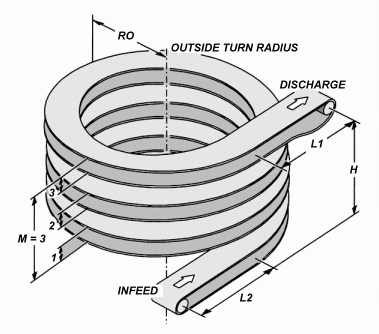FORMULA : TWS = TB × FA TWS in this unit means the tension calculation of spiral conveyor. FORMULA : TB = [ 2 × RO × M + ( L1 + L2 ) ] ( WP + 2WB ) × FBW + ( WP × H ) FORMULA : TA = BS × FS × FT Please refer to Table FT and Table FS.

Practical Example

The comparison of TA and TB, and other related calculations are the same as other types of conveyors. There are certain restrictions and regulations on the design and construction of the spiral conveyor. Therefore, while applying HONGSBELT spiral or turning belts to spiral conveyor system, we recommend you to refer to HONGSBELT Engineering manual and contact with our technical service department for further information and details.

Unit Tension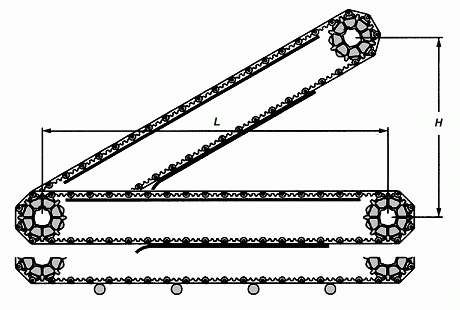FORMULA : TB = [ ( WP + 2WB ) X FBW ] X L + ( WP X H )

If carrying products are with the characteristic of piling up, the friction force Wf  that increases during the piling up conveyance should be subsume to the calculation.

 FORMULA : TB = [ ( WP + 2WB ) X FBW + Wf ] X L + ( WP X H ) FORMULA : Wf = WP X FBP X PP

Allowable Tension

Due to the different material of belt has different tensile strength that will be affected by temperature variation. Therefore, the calculation of unit allowable tension TA can be used to contrast with the belt total tension TW. This calculation result will help you to make the right choice of belt selection and match the demands of the conveyor. Please refer to Table FS and Table Ts in left menu.

 FORMULA : TA = BS X FS X FT BS = Conveyor Belt Tensile Strength ( Kg / M ) FS and FT Refer to Table FS and Table FT

Table Fs

Series HS-100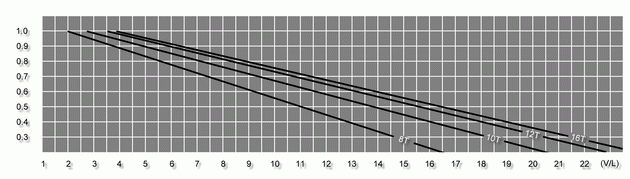Series HS-200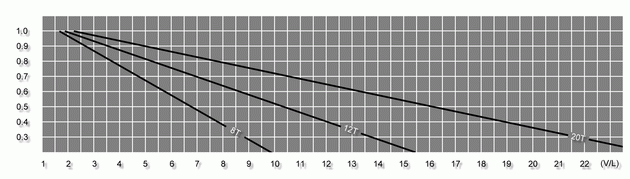Series HS-300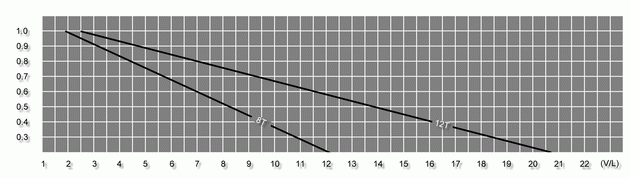Series HS-400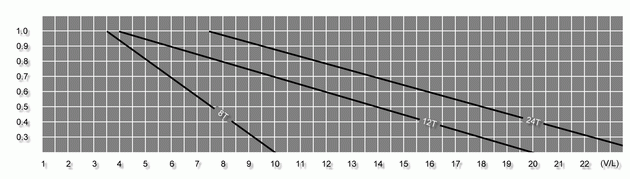Series HS-500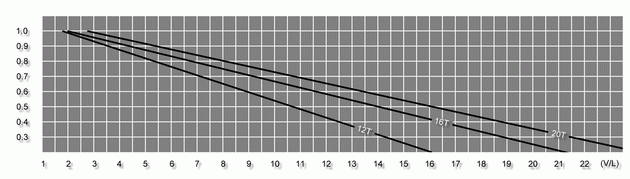Table Ts

Acetal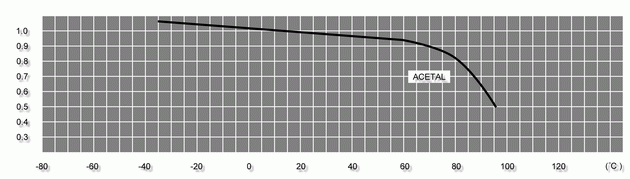Nylon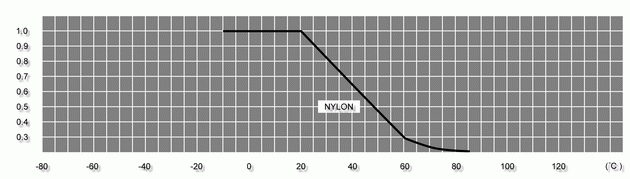Polyethylene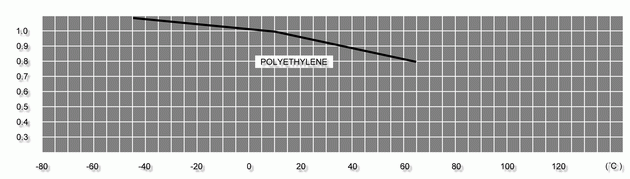Polypropylene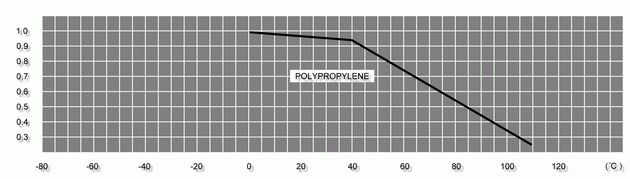Shaft Selection

 FORMULA : SL = ( TW + SW ) ?BW

Driven / Idler Shaft Weight Table - SW

 Shaft Dimensions Shaft Weight ( Kg/M ) Carbon Steel Stainless Steel Aluminum Alloy Square shaft 38mm 11.33 11.48 3.94 50mm 19.62 19.87 6.82 Round Shaft 30mm?/FONT> 5.54 5.62 1.93 45mm?/FONT> 12.48 12.64 4.34

Deflection of Drive / Idler Shaft - DS

Without Intermediate Bearing

 FORMULA : DS = 5 ?10-4 ( SL ?SB3 / E ?/FONT> I )

With Intermediate Bearing

 FORMULA : DS = 1 ?10-4 ( SL ?SB3 / E ?I )

Elasticity of Drive Shaft  - E

 Unit : Kg/mm2 Material Stainless Steel Carbon Steel Aluminum  Alloy Drive Shaft Elastic Rate 19700 21100 7000

Inertia Moment  - I

 Bore diameter of drive sprocket Inertia moment of shaft  ( mm4 ) Square Shaft 38mm 174817 50mm 1352750 Round Shaft 30mm?/FONT> 40791 45mm?/FONT> 326741

Drive Shaft Torque Calculation - TS

 FORMULA : TS = TW ?BW ?R

For the calculation value above, please compare with the table below for selecting the best drive shaft. If the torque of the drive shaft is still too strong, the smaller sprocket can be used to reduce the torque, and also economize the prime cost of shaft and bearing.

Using the smaller sprocket to fit the drive shaft that with the bigger diameter to reduce the torque, or using the bigger sprocket to fit the drive shaft that with  the smaller diameter to increase torque.

Maximum Torque Factor for Drive Shaft

 Torque Material Journal Diameter (mm) 50 45 40 35 30 25 20 Kg-mm x 1000 Stainless Steel 180 135 90 68 45 28 12 Carbon Steel 127 85 58 45 28 17 10 Aluminum Alloy -- -- -- 28 17 12 5

Horsepower

If the drive motor is selected for a gear reducer motor, the horsepower ratio should be greater than the carrying products and the total tensile force that generates during the belt running.

Horse Power (HP)

 FORMULA : = 2.2 × 10-4 × TW × BW × V = 2.2 × 10-4 ( TS × V / R ) = Watts × 0.00134

Watts

 FORMULA : = ( TW × BW × V ) / ( 6.12 × R ) = ( TS × V ) / ( 6.12 × R ) = HP × 745.7

Table FC

 Rail Material Temperature FC Belt Material Dry Wet HDPE / UHMW -10°C ~ 80°C P.P. 0.10 0.10 P.E. 0.30 0.20 Actel 0.10 0.10 Nylon 0.35 0.25 Acetal -10°C ~ 100°C P.P. 0.10 0.10 P.E. 0.10 0.10 Actel 0.10 0.10 Nylon 0.20 0.20

Please contrast the rails material and belt material of the conveyor with the transporting procedure in dry or wet environment to get value FC.

Ca, Cb Value

 Conveyor Belt Turning Angle Friction Coefficient Between Conveyor  Belt Edge & Rail Strip FC ≤ 0.15 FC ≤ 0.2 FC ≤ 0.3 Ca Cb Ca Cb Ca Cb ≥ 15 ° 1.04 0.023 1.05 0.021 1.00 0.023 ≥ 30 ° 1.08 0.044 1.11 0.046 1.17 0.048 ≥ 45 ° 1.13 0.073 1.17 0.071 1.27 0.075 ≥ 60 ° 1.17 0.094 1.23 0.096 1.37 0.10 ≥ 90 ° 1.27 0.15 1.37 0.15 1.6 0.17 ≥ 180 ° 1.6 0.33 1.88 0.37 2.57 0.44

After getting value FC from Table FC, please contrast it with the curved angle of the conveyor, and you can get value Ca and value Cb.

Service And Support
News And Events
Career